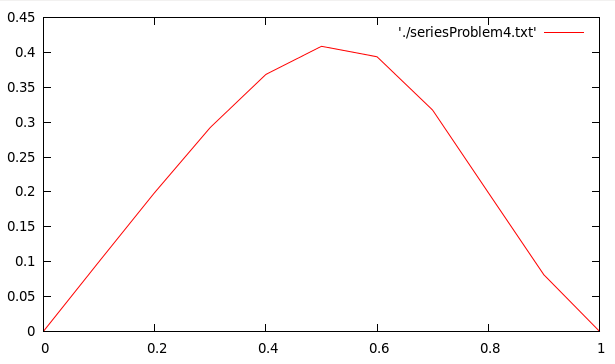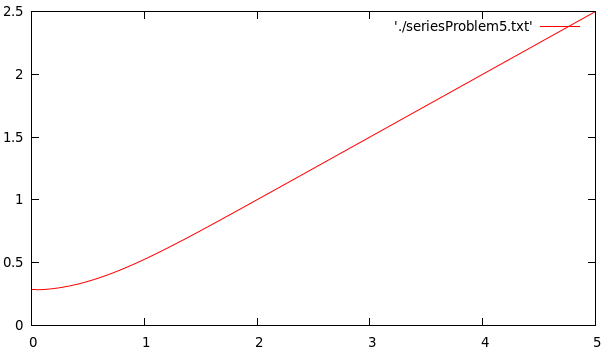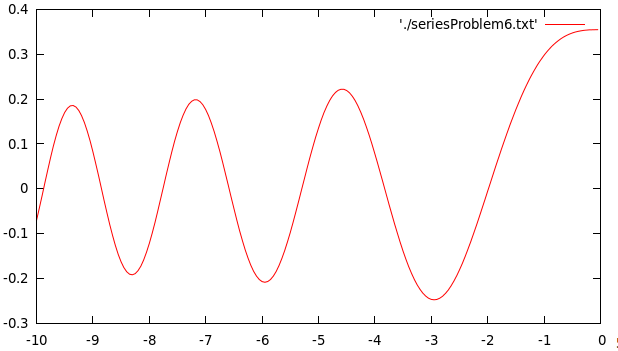# Problems on Infinite Series Sum – C PROGRAMMING

In my recent posts I have showed you how to write C programs that calculate the sum of a finite as well as an infinite series.

Now, that we have discussed a few examples like the Sine, Cosine and the Bessel series, we can try some harder problems.

In this post we will discuss three more series.

### Q. Evaluate$F(z)$ given by$F(z)=\cos \left(\frac{\pi z^2}{2}\right)\sum_{n=0}^\infty \frac{(-1)^n \pi^{2n}z^{4n+1}}{1.5.9....(4n+1)}$ correct to four decimal places, for$0 \leq z \leq 1$, at intervals of 0.1.

Solution.
The first term of the series is:$t_0=z$
and the ratio of the consecutive terms is:$R=\frac{t_i}{t_{i-1}}=-\frac{\pi^2z^4}{4i+1}$

### PROGRAM:

/*****************************
******SERIES PROBLEM*********
****************************/
#include<stdio.h>
#include<math.h>
main(){
FILE *fp=NULL;
fp=fopen("seriesProblem4.txt","w");
double t0,t1,R,sum,z,eps;
printf("Enter the desired accuracy: ");
scanf("%lf",&eps);
for(z=0;z<=1;z=z+0.1){
int i=1;
//Initialize First Term
t0=z;
//Make sum equal to the first term
sum=t0;
do{
//Find the ratio of the second term to the first term using already known relation
R=-(M_PI*M_PI*pow(z,4))/((4*i+1));
//Calculate the second term
t1=R*t0;
//find the new sum
sum=sum+t1;
t0=t1;
i++;
//keep on summing terms until the required accuracy is reached
}while(fabs(t1/sum)>eps);
sum=sum*cos(M_PI*z*z/2);
fprintf(fp,"%lf\t%lf\n",z,sum);
}

}


### OUTPUT:

When you run the above program, it will just ask you to enter the desired accuracy, create a file called 'seriesProblem4.txt' containing the data-points in the given range of x. You can then plot this file using GnuPlot by giving the command:
->plot 'seriesProblem4.txt' w l
The output looks something like this:### Q. Write a program to plot the sum of the following series:$f(z,n)=\sum_{k=0,2,4..}^{\infty}\frac{z^k}{2^{n-k}k!\Gamma (\frac{1}{2}+\frac{n-k}{2})}$ for$n=2$ and$z$ in the range$0 \leq z \leq 5$. You would require the following relations:$\Gamma (1/2)=\sqrt{\pi}$$\Gamma (z+1)=z\Gamma(z)$

Solution.
The first term of the series is:$t_0=\frac{1}{2\sqrt{\pi}}$
and the ratio of the consecutive terms is:$R=\frac{t_i}{t_{i-1}}=\frac{4z^2(3-k)}{2k(k-1)}$

### PROGRAM:

/*****************************
******SERIES PROBLEM*********
****************************/
#include<stdio.h>
#include<math.h>
main(){
FILE *fp=NULL;
fp=fopen("seriesProblem5.txt","w");
double t0,t1,R,sum,z,eps;
printf("Enter the desired accuracy: ");
scanf("%lf",&eps);
for(z=0;z<=5;z=z+0.01){
int k=2;
//Initialize First Term
t0=1/(2*sqrt(M_PI));
//Make sum equal to the first term
sum=t0;
do{
//Find the ratio of the second term to the first term using already known relation
R=(4*z*z*(3-k))/(2*k*(k-1));
//Calculate the second term
t1=R*t0;
//find the new sum
sum=sum+t1;
t0=t1;
k=k+2;
//keep on summing terms until the required accuracy is reached
}while(fabs(t1/sum)>eps);
fprintf(fp,"%lf\t%lf\n",z,sum);
}
}


### OUTPUT:

When you run the above program, it will just ask you to enter the desired accuracy, create a file called 'seriesProblem5.txt' containing the data-points in the given range of x. You can then plot this file using GnuPlot by giving the command:
->plot 'seriesProblem5.txt' w l
The output looks something like this:### Q. Write a program to plot the following function:$f(z)=C\left(1+\frac{z^3}{3!}+\frac{1.4.z^6}{6!}+\frac{1.4.7.z^9}{9!}+....\right)$where$C=0.35503$, for$z$ in the range$-10 \leq z \leq 0$, at intervals of 0.05.

Solution.

The first term of the series is:$t_0=1$
and the ratio of the consecutive terms is:$R=\frac{t_i}{t_{i-1}}=\frac{z^3}{3i(3i-1)}$

### PROGRAM:

/*****************************
******SERIES PROBLEM*********
****************************/
#include<stdio.h>
#include<math.h>
main(){
FILE *fp=NULL;
fp=fopen("seriesProblem6.txt","w");
double t0,t1,R,sum,z,eps;
printf("Enter the desired accuracy: ");
scanf("%lf",&eps);
for(z=-10;z<=0;z=z+0.05){
int i=1;
//Initialize First Term
t0=1;
//Make sum equal to the first term
sum=t0;
do{
//Find the ratio of the second term to the first term using already known relation
R=pow(z,3)/((3*i)*(3*i-1));
//Calculate the second term
t1=R*t0;
//find the new sum
sum=sum+t1;
t0=t1;
i++;
//keep on summing terms until the required accuracy is reached
}while(fabs(t1/sum)>eps);
sum=sum*0.35503;
fprintf(fp,"%lf\t%lf\n",z,sum);
}

}



### OUTPUT:

When you run the above program, it will just ask you to enter the desired accuracy, create a file called 'seriesProblem6.txt' containing the data-points in the given range of x. You can then plot this file using GnuPlot by giving the command:
->plot 'seriesProblem6.txt' w l
The output looks something like this:Solution:

### PROGRAM:

/********************************
******FINITE SERIES SUM**********
Series: S(x) = 1 + (1/x^2) + (1/x^3) + ..... + (1/x^n)
********************************/
#include<stdio.h>
#include<math.h>
main(){
FILE *fp=NULL;
fp=fopen("seriesProblem1.txt","w");
int i,n;
printf("Enter the number of terms to be summed(n): ");
scanf("%d",&n);
double x,xmin,xmax;
printf("Enter the the range of x:\nxmin = ");
scanf("%lf",&xmin);
printf("xmax = ");
scanf("%lf",&xmax);
for(x=xmin;x<=xmax;x=x+0.1){
/*Initialize t0 with the value of the first term of the series */
double t0=1/(x*x);
double t1,R,sum=1+t0;
for(i=1;i<n-1;i++){
R=(double)1/x;
t1=R*t0;
t0=t1;
sum=sum+t1;
}
fprintf(fp,"%lf\t%lf\n",x,sum);
}
}


### REFERENCES:

The above problems have been taken from the Computer Programming & Numerical Analysis Manual by Dr. Shobhit Mahajan.